Start of section Production, amateur Radio amateurs Aircraft model, rocket-model Useful, entertaining Stealth Master Electronics Physics Technologies Inventions Secrets of the cosmos Secrets of the Earth Secrets of the Ocean Tricks Map of section Use of the site materials is allowed subject to the link (for websites - hyperlinks)

# SUN - BLACK HOLE KERA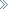Leave a comment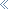Introduction

For fifty years after the creation of the general theory of relativity, only Schwarzschild's solution describing a spherically symmetric black hole characterized by mass only was used in all calculations. The idea that sufficiently realistic models of black holes must have rotation is not new. Everyone understood that it was necessary to take into account the effect of rotation, but no one could correctly solve the rotation should depend on two parameters - the mass of the black hole (denoted by the letter M) of the Einstein equation. Strictly speaking, a complete solution of the gravitational field equations taking into account the angular momentum of the hole (denoted by a). In addition, this solution must be asymptotically flat, that is, away from the black hole, space-time should become flat. Although flat space never happens, but at a certain distance - up to the observed effect of a black hole, it can be assumed that it is flat. Like all assumptions in mathematical modeling, rejecting them leads to a revision of the entire model. Therefore, the assumption of an asymptotically flat space in mathematical models of black holes remains so far, so it does not have a significant effect on the structure of a black hole, but it turns out to be unacceptable when considering a model of space in which a black hole is an element of a more complex structure. The equations of the gravitational field turned out to be so complicated mathematically that no one could find a single exact solution for a long time that satisfied these simple requirements. Only in 1963, Roy P. Kerr, an Australian mathematician who then worked at the University of Texas (USA), found a complete solution of the gravitational field equations for a rotating black hole. For the first time almost fifty years after Einstein's fundamental work, astrophysicists finally obtained a mathematical description of the geometry of space-time surrounding a massive rotating object. By 1975, the uniqueness of the Kerr solution was proved. In exactly the same way as all possible solutions for black holes with only a mass (M) are equivalent to the Schwarzschild solution, and all possible solutions for black holes with mass and charge (M and Q) are equivalent to the Riesner-Nordström solution, all possible solutions with mass And the angular momentum (M and a) must be equivalent to the Kerr solution.

SOLUTION OF SOLUTION OF EQUATIONS FIELD DESCRIBING BLACK HOLES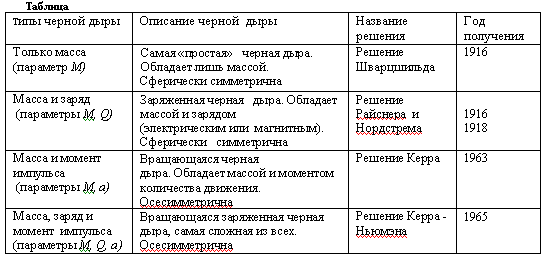The sun has a mass of - M and momentum - and therefore in the future we will consider the structure of the Sun in the Kerr solution.

Authors: Gordeev SI, Voloshina VN 28-07-2003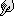NEW ARTICLES AND PUBLICATIONSThe technology of manufacturing universal couplings for unbreakable, threadless, flossless connection of pipe sections in high pressure pipelines (video is available )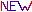Technology of oil and oil products cleaningOn the possibility of moving a closed mechanical system at the expense of internal forcesFluorescence in thin dielectric channelsThe relationship between quantum and classical mechanicsMillimeter waves in medicine. A New Look. MMV therapyMagnetic engineHeat source based on pump units